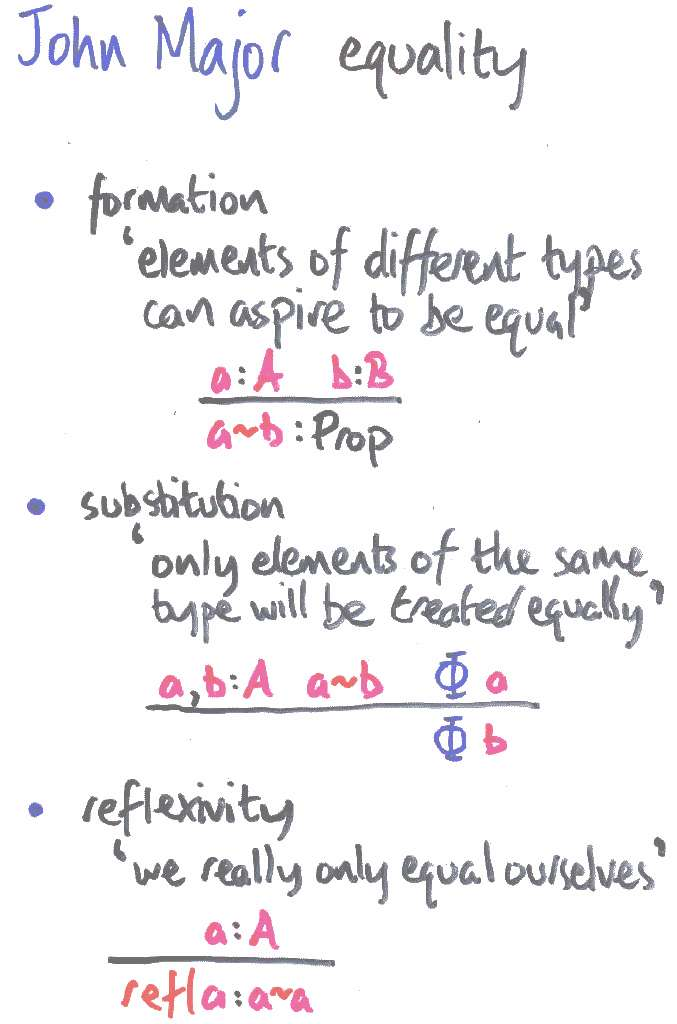Next: lifting a finite mapping | Prev: Henry Ford generalisation | Up: contentsSeasoned observers will notice that the substitution rule is not the usual elimination rule you'd get if you defined ~ inductively. But you can derive it...if you have conventional equality plus the Altenkirch-Streicher `uniqueness of identity proofs' axiom, which Hofmann showed was not derivable in ye olde type theory.

Alternatively, you can see ~ as conventional equality for dependent pairs of a type and a term which inhabits it. Then substitutivity for ~ is an instance of (A,a)=(A,b) -> a=b, which is notoriously equivalent to uniqueness of identity proofs.

If you have ~ as fundamental, then you can define = inductively (a la Paulin-Mohring, par exemple), then derive uniqueness of identity proofs.

So it's all the same deal, more or less...

The effect of the equational generalisation is to reflect at the object level the typechecking of patterns. Because only well-typed terms get evaluated, the LEGO terms generated in this way only ever introduce and eliminate reflexive equations. As far as program execution is concerned, the equational reasoning is transient. The pattern equations really are true at the level of conversion: they are admissable as reductions, leaving the equational phase to the typechecker. We may regard pattern-matching programs so produced as refreshingly free of propositional baggage.

Next: lifting a finite mapping | Prev: Henry Ford generalisation | Up: contents

Conor's Work Page